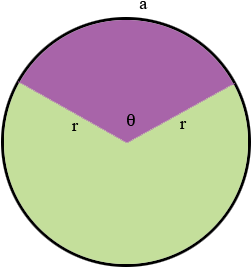SEARCH HOMEMath Central Quandaries & QueriesQuestion from mustafa, a student: In a sector of a circle, the arc length is equal to half the perimeter of a sector. Find the area of a sector in terms of rHi,

Suppose the length of the arc is $a$ units and the measure of the angle is $\theta$ units. You can use degrees or radians, it's your choice.Write $a$ in terms of $a$ and $r.$

The perimeter of the sector is $a + 2r.$ Since the length of the arc is half the perimeter you have a equation relating $r$ and $\theta.$ Solve for $\theta.$

What is the area of the sector?

PennyMath Central is supported by the University of Regina and the Imperial Oil Foundation.